# Comparison between evidence (Dempster-Shafer) theory and Bayesian theory

Both Bayesian theory and Dempster-Shafer (evidence) theory assign non-negative weights to set of events. In Bayesian theory, the finite set of possible events denoted by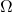, each individual event,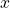is assigned a non-negative weight called probability denoted by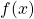. The probabilities satisfy the following properties.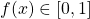for all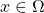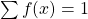In Dempster-Shafer theory, the finite set called frame of discernment,comprises all possible combination of events. Therefore, any subset of the frame of discernment can be assigned a non-negative weight called mass denoted by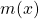. The masses satisfy the following properties.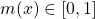for all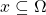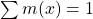and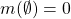where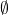is the empty set.

For example, a coin of two sides has two possible outcomes (events): head and tail. A Bayesian approach assigns probability individually to head and tail as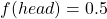and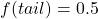. DS theory would assign mass to each of the possibilities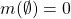,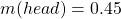,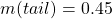and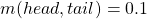. This allows DS theory to explicitly represent an ignorance or ambiguity (uncertainty) about the situation being observed. Refer ,  for more information about comparison of Bayesian theory and Dempster-Shafer theory.

 J. C. Hoffman and R. R. Murphy, “Comparison of Bayesian and Dempster-Shafer Theory for Sensing: A Practitioner’s Approach,” in in SPIE Proc. on Neural and Stochastic Methods in Image and Signal Processing II, 1993, pp. 266–279.
 P. Soundappan, E. Nikolaidis, R. T. Haftka, R. Grandhi, and R. Canfield, “Comparison of evidence theory and Bayesian theory for uncertainty modeling,” Reliability Engineering & System Safety, vol. 85, no. 1–3, pp. 295–311, Jul. 2004.